# Keras learning rate schedules and decay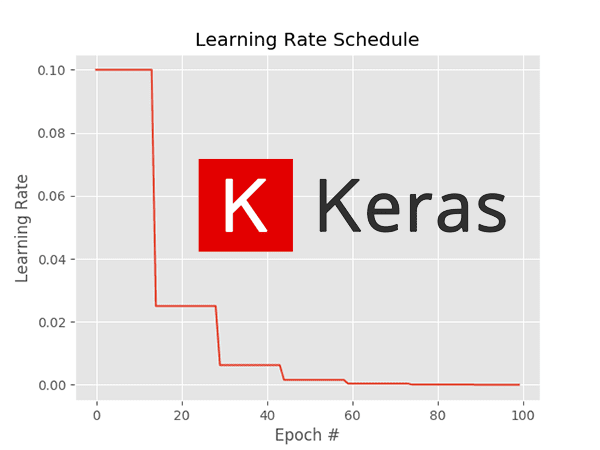In this tutorial, you will learn about learning rate schedules and decay using Keras. You’ll learn how to use Keras’ standard learning rate decay along with step-based, linear, and polynomial learning rate schedules.

When training a neural network, the learning rate is often the most important hyperparameter for you to tune:

• Too small a learning rate and your neural network may not learn at all
• Too large a learning rate and you may overshoot areas of low loss (or even overfit from the start of training)

When it comes to training a neural network, the most bang for your buck (in terms of accuracy) is going to come from selecting the correct learning rate and appropriate learning rate schedule.

But that’s easier said than done.

To help deep learning practitioners such as yourself learn how to assess a problem and choose an appropriate learning rate, we’ll be starting a series of tutorials on learning rate schedules, decay, and hyperparameter tuning with Keras.

By the end of this series, you’ll have a good understanding of how to appropriately and effectively apply learning rate schedules with Keras to your own deep learning projects.

To learn how to use Keras for learning rate schedules and decay, just keep reading

Looking for the source code to this post?

## Keras learning rate schedules and decay

In the first part of this guide, we’ll discuss why the learning rate is the most important hyperparameter when it comes to training your own deep neural networks.

We’ll then dive into why we may want to adjust our learning rate during training.

From there I’ll show you how to implement and utilize a number of learning rate schedules with Keras, including:

• The decay schedule built into most Keras optimizers
• Step-based learning rate schedules
• Linear learning rate decay
• Polynomial learning rate schedules

We’ll then perform a number of experiments on the CIFAR-10 using these learning rate schedules and evaluate which one performed the best.

These sets of experiments will serve as a template you can use when exploring your own deep learning projects and selecting an appropriate learning rate and learning rate schedule.

### Why adjust our learning rate and use learning rate schedules?

To see why learning rate schedules are a worthwhile method to apply to help increase model accuracy and descend into areas of lower loss, consider the standard weight update formula used by nearly all neural networks: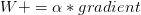$W += \alpha * gradient$

Recall that the learning rate,$\alpha$, controls the “step” we make along the gradient. Larger values of$\alpha$ imply that we are taking bigger steps. While smaller values of$\alpha$ will make tiny steps. If$\alpha$ is zero the network cannot make any steps at all (since the gradient multiplied by zero is zero).

Most initial learning rates (but not all) you encounter are typically in the set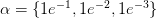$\alpha = \{1e^{-1}, 1e^{-2}, 1e^{-3}\}$ .

A network is then trained for a fixed number of epochs without changing the learning rate.

This method may work well in some situations, but it’s often beneficial to decrease our learning rate over time. When training our network, we are trying to find some location along our loss landscape where the network obtains reasonable accuracy. It doesn’t have to be a global minima or even a local minima, but in practice, simply finding an area of the loss landscape with reasonably low loss is “good enough”.

If we constantly keep a learning rate high, we could overshoot these areas of low loss as we’ll be taking too large of steps to descend into those series.

Instead, what we can do is decrease our learning rate, thereby allowing our network to take smaller steps — this decreased learning rate enables our network to descend into areas of the loss landscape that are “more optimal” and would have otherwise been missed entirely by our learning rate learning.

We can, therefore, view the process of learning rate scheduling as:

1. Finding a set of reasonably “good” weights early in the training process with a larger learning rate.
2. Tuning these weights later in the process to find more optimal weights using a smaller learning rate.

We’ll be covering some of the most popular learning rate schedules in this tutorial.

### Project structure

Once you’ve grabbed and extracted the “Downloads” go ahead and use the tree  command to inspect the project folder:

Our output/  directory will contain learning rate and training history plots. The five experiments included in the results section correspond to the five plots with the train_*.png  filenames, respectively.

The pyimagesearch  module contains our ResNet CNN and our learning_rate_schedulers.py . The LearningRateDecay  parent class simply includes a method called plot  for plotting each of our types of learning rate decay. Also included are subclasses, StepDecay  and PolynomialDecay  which calculate the learning rate upon the completion of each epoch. Both of these classes contain the plot  method via inheritance (an object-oriented concept).

Our training script, train.py , will train ResNet on the CIFAR-10 dataset. We’ll run the script with the absence of learning rate decay as well as standard, linear, step-based, and polynomial learning rate decay.

### The standard “decay” schedule in Keras

The Keras library ships with a time-based learning rate scheduler — it is controlled via the decay  parameter of the optimizer class (such as SGD, Adam, etc.).

To discover how we can utilize this type of learning rate decay, let’s take a look at an example of how we may initialize the ResNet architecture and the SGD optimizer:

Here we initialize our SGD optimizer with an initial learning rate of 1e-2 . We then set our decay  to be the learning rate divided by the total number of epochs we are training the network for (a common rule of thumb).

Internally, Keras applies the following learning rate schedule to adjust the learning rate after every batch update — it is a misconception that Keras updates the standard decay after every epoch. Keep this in mind when using the default learning rate scheduler supplied with Keras.

The update formula follows: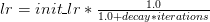$lr = init\_lr * \frac{1.0}{1.0 + decay * iterations}$

Using the CIFAR-10 dataset as an example, we have a total of 50,000 training images.

If we use a batch size of 64 , that implies there are a total of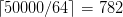$\lceil50000 / 64\rceil = 782$ steps per epoch. Therefore, a total of 782  weight updates need to be applied before an epoch completes.

To see an example of the learning rate schedule calculation, let’s assume our initial learning rate is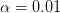$\alpha = 0.01$ and our$decay = \frac{0.01}{40}$ (with the assumption that we are training for forty epochs).

The learning rate at step zero, before any learning rate schedule has been applied, is: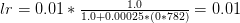$lr = 0.01 * \frac{1.0}{1.0 + 0.00025 * (0 * 782)} = 0.01$

At the beginning of epoch one we can see the following learning rate: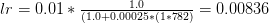$lr = 0.01 * \frac{1.0}{(1.0 + 0.00025 * (1 * 782)} = 0.00836$

Figure 1 below continues the calculation of Keras’ standard learning rate decay$\alpha =0.01$ and a decay of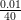$\frac{0.01}{40}$:

You’ll learn how to utilize this type of learning rate decay inside the “Implementing our training script” and “Keras learning rate schedule results” sections of this post, respectively.

### Our LearningRateDecay class

In the remainder of this tutorial, we’ll be implementing our own custom learning rate schedules and then incorporating them with Keras when training our neural networks.

To keep our code neat and tidy, and not to mention, follow object-oriented programming best practices, let’s first define a base LearningRateDecay  class that we’ll subclass for each respective learning rate schedule.

Open up the learning_rate_schedulers.py  in your directory structure and insert the following code:

Each and every learning rate schedule we implement will have a plot function, enabling us to visualize our learning rate over time.

With our base LearningRateSchedule  class implement, let’s move on to creating a step-based learning rate schedule.

### Step-based learning rate schedules with Keras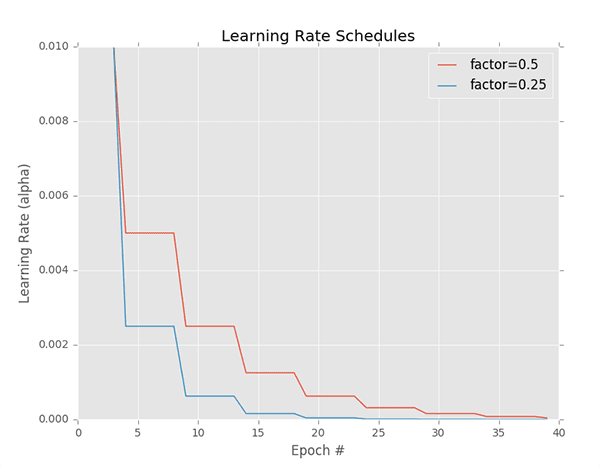Figure 2: Keras learning rate step-based decay. The schedule in red is a decay factor of 0.5 and blue is a factor of 0.25.

One popular learning rate scheduler is step-based decay where we systematically drop the learning rate after specific epochs during training.

The step decay learning rate scheduler can be seen as a piecewise function, as visualized in Figure 2 — here the learning rate is constant for a number of epochs, then drops, is constant once more, then drops again, etc.

When applying step decay to our learning rate, we have two options:

1. Define an equation that models the piecewise drop-in learning rate that we wish to achieve.
2. Use what I call the ctrl + c method to train a deep neural network. Here we train for some number of epochs at a given learning rate and eventually notice validation performance stagnating/stalling, then  ctrl + c to stop the script, adjust our learning rate, and continue training.

We’ll primarily be focusing on the equation-based piecewise drop to learning rate scheduling in this post.

The ctrl + c method is a bit more advanced and normally applied to larger datasets using deeper neural networks where the exact number of epochs required to obtain a reasonable model is unknown.

If you’d like to learn more about the ctrl + c method to training, please refer to Deep Learning for Computer Vision with Python.

When applying step decay, we often drop our learning rate by either (1) half or (2) an order of magnitude after every fixed number of epochs. For example, let’s suppose our initial learning rate is$\alpha = 0.01$.

After 10 epochs we drop the learning rate to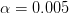$\alpha = 0.005$.

After another 10 epochs (i.e., the 20th total epoch),$\alpha$ is dropped by a factor of 0.5  again, such that$\alpha = 0.0025$, etc.

In fact, this is the exact same learning rate schedule that is depicted in Figure 2 (red line).

The blue line displays a more aggressive drop factor of 0.25 . Modeled mathematically, we can define our step-based decay equation as: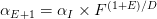$\alpha_{E + 1} = \alpha_{I} \times F^{(1 + E) / D}$

Where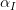$\alpha_{I}$ is the initial learning rate,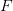$F$ is the factor value controlling the rate in which the learning date drops, D is the “Drop every” epochs value, and E is the current epoch.

The larger our factor$F$ is, the slower the learning rate will decay.

Conversely, the smaller the factor$F$, the faster the learning rate will decay.

All that said, let’s go ahead and implement our StepDecay  class now.

Go back to your learning_rate_schedulers.py  file and insert the following code:

Line 20 defines the constructor to our StepDecay  class. We then store the initial learning rate ( initAlpha ), drop factor, and dropEvery  epochs values (Lines 23-25).

The __call__ function:

• Accepts the current epoch  number.
• Computes the learning rate based on the step-based decay formula detailed above (Lines 29 and 30).
• Returns the computed learning rate for the current epoch (Line 33).

You’ll see how to use this learning rate schedule later in this post.

### Linear and polynomial learning rate schedules in Keras

Two of my favorite learning rate schedules are linear learning rate decay and polynomial learning rate decay.

Using these methods our learning rate is decayed to zero over a fixed number of epochs.

The rate in which the learning rate is decayed is based on the parameters to the polynomial function. A smaller exponent/power to the polynomial will cause the learning rate to decay “more slowly”, whereas larger exponents decay the learning rate “more quickly”.

Conveniently, both of these methods can be implemented in a single class:

Line 36 defines the constructor to our PolynomialDecay  class which requires three values:

• maxEpochs : The total number of epochs we’ll be training for.
• initAlpha : The initial learning rate.
• power : The power/exponent of the polynomial.

Note that if you set power=1.0  then you have a linear learning rate decay.

Lines 45 and 46 compute the adjusted learning rate for the current epoch while Line 49 returns the new learning rate.

### Implementing our training script

Now that we’ve implemented a few different Keras learning rate schedules, let’s see how we can use them inside an actual training script.

Create a file named  train.py  file in your editor and insert the following code:

Lines 2-16 import required packages. Line 3 sets the matplotlib  backend so that we can create plots as image files. Our most notable imports include:

• StepDecay : Our class which calculates and plots step-based learning rate decay.
• PolynomialDecay : The class we wrote to calculate polynomial-based learning rate decay.
• ResNet : Our Convolutional Neural Network implemented in Keras.
• LearningRateScheduler : A Keras callback. We’ll pass our learning rate schedule  to this class which will be called as a callback at the completion of each epoch to calculate our learning rate.

Let’s move on and parse our command line arguments:

Our script accepts any of four command line arguments when the script is called via the terminal:

• --schedule : The learning rate schedule method. Valid options are “standard”, “step”, “linear”, “poly”. By default, no learning rate schedule will be used.
• --epochs : The number of epochs to train for ( default=100 ).
• --lr-plot : The path to the output plot. I suggest overriding the default  of lr.png  with a more descriptive path + filename.
• --train-plot : The path to the output accuracy/loss training history plot. Again, I suggest a descriptive path + filename, otherwise training.png  will be set by default .

With our imports and command line arguments in hand, now it’s time to initialize our learning rate schedule:

Line 33 sets the number of epochs  we will train for directly from the command line args  variable. From there we’ll initialize our callbacks  list and learning rate schedule  (Lines 34 and 35).

Lines 38-50 then select the learning rate schedule  if args["schedule"]  contains a valid value:

• "step" : Initializes StepDecay .
• "linear" : Initializes PolynomialDecay  with power=1  indicating that a linear learning rate decay will be utilized.
• "poly" :  PolynomialDecay  with a power=5  will be used.

After you’ve reproduced the results of the experiments in this tutorial, be sure to revisit Lines 38-50 and insert additional elif  statements of your own so you can run some of your own experiments!

Lines 54 and 55 initialize the LearningRateScheduler  with the schedule as a single callback part of the callbacks  list. There is a case where no learning rate decay will be used (i.e. if the --schedule  command line argument is not overridden when the script is executed).

Line 60 loads our CIFAR-10 data. The dataset is conveniently already split into training and testing sets.

The only preprocessing we must perform is to scale the data into the range [0, 1] (Lines 61 and 62).

Lines 65-67 binarize the labels and then Lines 70 and 71 initialize our labelNames  (i.e. classes). Do not add to or alter the labelNames  list as order and length of the list matter.

Let’s initialize decay parameter:

Line 74 initializes our learning rate decay .

If we’re using the "standard"  learning rate decay schedule, then the decay is initialized as 1e-1 / epochs  (Lines 78-80).

With all of our initializations taken care of, let’s go ahead and compile + train our ResNet  model:

Our Stochastic Gradient Descent ( SGD ) optimizer is initialized on Line 87 using our decay .

From there, Lines 88 and 89 build our ResNet  CNN with an input shape of 32x32x3 and 10 classes. For an in-depth review of ResNet, be sure refer to Chapter 10: ResNet of Deep Learning for Computer Vision with Python.

Our model  is compiled with a loss  function of "categorical_crossentropy"  since our dataset has > 2 classes. If you use a different dataset with only 2 classes, be sure to use loss="binary_crossentropy" .

Lines 94 and 95 kick of our training process. Notice that we’ve provided the callbacks  as a parameter. The callbacks  will be called when each epoch is completed. Our LearningRateScheduler  contained therein will handle our learning rate decay (so long as callbacks  isn’t an empty list).

Finally, let’s evaluate our network and generate plots:

Lines 99-101 evaluate our network and print a classification report to our terminal.

Lines 104-115 generate and save our training history plot (accuracy/loss curves). Lines 119-121 generate a learning rate schedule plot, if applicable. We will inspect these plot visualizations in the next section.

### Keras learning rate schedule results

With both our (1) learning rate schedules and (2) training scripts implemented, let’s run some experiments to see which learning rate schedule will perform best given:

1. An initial learning rate of 1e-1
2. Training for a total of 100  epochs

#### Experiment #1: No learning rate decay/schedule

As a baseline, let’s first train our ResNet model on CIFAR-10 with no learning rate decay or schedule: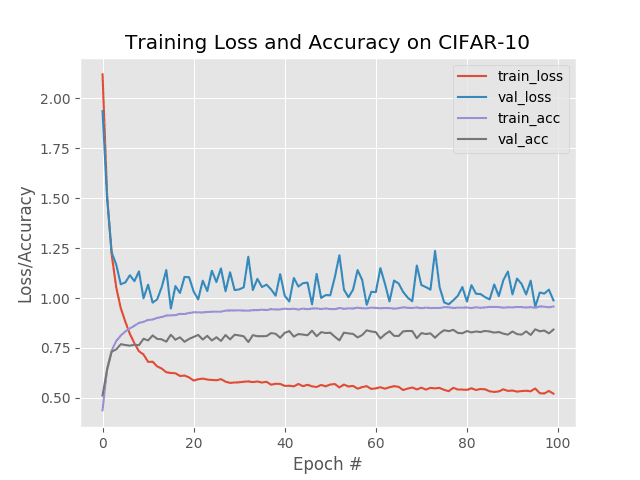Figure 3: Our first experiment for training ResNet on CIFAR-10 does not have learning rate decay.

Here we obtain ~85% accuracy, but as we can see, validation loss and accuracy stagnate past epoch ~15 and do not improve over the rest of the 100 epochs.

Our goal is now to utilize learning rate scheduling to beat our 85% accuracy (without overfitting).

#### Experiment: #2: Keras standard optimizer learning rate decay

In our second experiment we are going to use Keras’ standard decay-based learning rate schedule:Figure 4: Our second learning rate decay schedule experiment uses Keras’ standard learning rate decay schedule.

This time we only obtain 82% accuracy, which goes to show, learning rate decay/scheduling will not always improve your results! You need to be careful which learning rate schedule you utilize.

#### Experiment #3: Step-based learning rate schedule results

Let’s go ahead and perform step-based learning rate scheduling which will drop our learning rate by a factor of 0.25 every 15 epochs: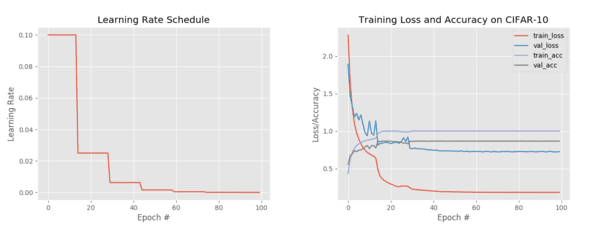Figure 5: Experiment #3 demonstrates a step-based learning rate schedule (left). The training history accuracy/loss curves are shown on the right.

Figure 5 (left) visualizes our learning rate schedule. Notice how after every 15 epochs our learning rate drops, creating the “stair-step”-like effect.

Figure 5 (right) demonstrates the classic signs of step-based learning rate scheduling — you can clearly see our:

1. Training/validation loss decrease
2. Training/validation accuracy increase

…when our learning rate is dropped.

This is especially pronounced in the first two drops (epochs 15 and 30), after which the drops become less substantial.

This type of steep drop is a classic sign of a step-based learning rate schedule being utilized — if you see that type of training behavior in a paper, publication, or another tutorial, you can be almost sure that they used step-based decay!

Getting back to our accuracy, we’re now at 86-87% accuracy, an improvement from our first experiment.

#### Experiment #4: Linear learning rate schedule results

Let’s try using a linear learning rate schedule with Keras by setting  power=1.0 :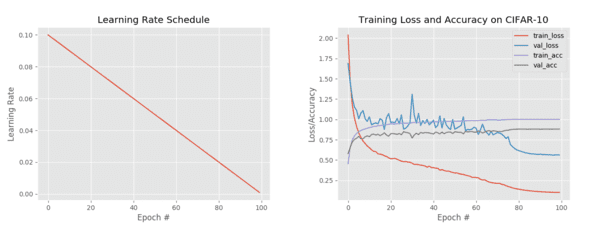Figure 6: Linear learning rate decay (left) applied to ResNet on CIFAR-10 over 100 epochs with Keras. The training accuracy/loss curve is displayed on the right.

Figure 6 (left) shows that our learning rate is decreasing linearly over time while Figure 6 (right) visualizes our training history.

We’re now seeing a sharper drop in both training and validation loss, especially past approximately epoch 75; however, note that our training loss is dropping significantly faster than our validation loss — we may be at risk of overfitting.

Regardless, we are now obtaining 88% accuracy on our data, our best result thus far.

#### Experiment #5: Polynomial learning rate schedule results

As a final experiment let’s apply polynomial learning rate scheduling with Keras by setting power=5 :

Figure 7 (left) visualizes the fact that our learning rate is now decaying according to our polynomial function while Figure 7 (right) plots our training history.

This time we obtain ~86% accuracy.

### Commentary on learning rate schedule experiments

Our best experiment was from our fourth experiment where we utilized a linear learning rate schedule.

But does that mean we should always use a linear learning rate schedule?

No, far from it, actually.

The key takeaway here is that for this:

• Particular dataset (CIFAR-10)
• Particular neural network architecture (ResNet)
• Initial learning rate of 1e-2
• Number of training epochs (100)

…is that linear learning rate scheduling worked the best.

No two deep learning projects are alike so you will need to run your own set of experiments, including varying the initial learning rate and the total number of epochs, to determine the appropriate learning rate schedule (additional commentary is included in the “Summary” section of this tutorial as well).

### Do other learning rate schedules exist?

Other learning rate schedules exist, and in fact, any mathematical function that can accept an epoch or batch number as an input and returns a learning rate can be considered a “learning rate schedule”. Two other learning rate schedules you may encounter include (1) exponential learning rate decay, as well as (2) cyclical learning rates.

I don’t often use exponential decay as I find that linear and polynomial decay are more than sufficient, but you are more than welcome to subclass the LearningRateDecay  class and implement exponential decay if you so wish.

Cyclical learning rates, on the other hand, are very powerful — we’ll be covering cyclical learning rates in a tutorial later in this series.

### How do I choose my initial learning rate?

You’ll notice that in this tutorial we did not vary our learning rate, we kept it constant at 1e-2 .

When performing your own experiments you’ll want to combine:

1. Learning rate schedules…
2. …with different learning rates

Don’t be afraid to mix and match!

The four most important hyperparameters you’ll want to explore, include:

1. Initial learning rate
2. Number of training epochs
3. Learning rate schedule
4. Regularization strength/amount (L2, dropout, etc.)

Finding an appropriate balance of each can be challenging, but through many experiments, you’ll be able to find a recipe that leads to a highly accurate neural network.

If you’d like to learn more about my tips, suggestions, and best practices for learning rates, learning rate schedules, and training your own neural networks, refer to my book, Deep Learning for Computer Vision with Python.

Today’s tutorial introduced you to learning rate decay and schedulers using Keras. To learn more about learning rates, schedulers, and how to write custom callback functions, refer to my book, Deep Learning for Computer Vision with Python.

Inside the book I cover:

1. More details on learning rates (and how a solid understanding of the concept impacts your deep learning success)
2. How to spot under/overfitting on-the-fly with a custom training monitor callback
3. How to checkpoint your models with a custom callback
4. My tips/tricks, suggestions, and best practices for training CNNs

Besides content on learning rates, you’ll also find:

• Super practical walkthroughs that present solutions to actual, real-world image classification, object detection, and instance segmentation problems.
• Hands-on tutorials (with lots of code) that not only show you the algorithms behind deep learning for computer vision but their implementations as well.
• A no-nonsense teaching style that is guaranteed to help you master deep learning for image understanding and visual recognition.

## Summary

In this tutorial, you learned how to utilize Keras for learning rate decay and learning rate scheduling.

Specifically, you discovered how to implement and utilize a number of learning rate schedules with Keras, including:

• The decay schedule built into most Keras optimizers
• Step-based learning rate schedules
• Linear learning rate decay
• Polynomial learning rate schedules

After implementing our learning rate schedules we evaluated each on a set of experiments on the CIFAR-10 dataset.

Our results demonstrated that for an initial learning rate of 1e-2 , the linear learning rate schedule, decaying over 100  epochs, performed the best.

However, this does not mean that a linear learning rate schedule will always outperform other types of schedules. Instead, all this means is that for this:

• Particular dataset (CIFAR-10)
• Particular neural network architecture (ResNet)
• Initial learning rate of 1e-2
• Number of training epochs ( 100 )

…that linear learning rate scheduling worked the best.

No two deep learning projects are alike so you will need to run your own set of experiments, including varying the initial learning rate, to determine the appropriate learning rate schedule.

I suggest you keep an experiment log that details any hyperparameter choices and associated results, that way you can refer back to it and double-down on experiments that look promising.

Do not expect that you’ll be able to train a neural network and be “one and done” — that rarely, if ever, happens. Instead, set the expectation with yourself that you’ll be running many experiments and tuning hyperparameters as you go along. Machine learning, deep learning, and artificial intelligence as a whole are iterative — you build on your previous results.

Later in this series of tutorials I’ll also be showing you how to select your initial learning rate.If you would like to download the code and images used in this post, please enter your email address in the form below. Not only will you get a .zip of the code, I’ll also send you a FREE 17-page Resource Guide on Computer Vision, OpenCV, and Deep Learning. Inside you'll find my hand-picked tutorials, books, courses, and libraries to help you master CV and DL! Sound good? If so, enter your email address and I’ll send you the code immediately!

### 31 Responses to Keras learning rate schedules and decay

1.David Bonn July 22, 2019 at 11:52 am #

As always, a fantastic post!

As you said, it is important to keep copious notes of your experiments which will make it easier to reproduce them or continue work when you come back to them later.

One good (and very simple) practice is to have your training script echo the hyperparameters (e.g. batch size, learning rate, learning rate schedule) to standard output. This makes it easier to figure out what you’re looking at when reviewing that output sometime later.

A lot of times you can dramatically decrease your cycle time by working with a random subset of your dataset. Once you’ve got a fairly good set of hyperparameters you can usually rapidly increase the dataset size and won’t have to make too many tweaks.

If you have multiple GPUs, running multiple experiments at the same time rather than have one experiment try to use all of the GPUs is usually a win. Especially how Keras scales so poorly with multiple GPUs. In a similar vein, I’ve considered (but not yet implemented) running multiple experiments on AWS through multiple instances at the same time.

I have tried cyclic learning rates. They appear very powerful but for the problems I was chasing they didn’t seem to help very much. This wasn’t really a matter of them working poorly but rather linear rate decay working extremely well. Like you said every dataset and model architecture is going to be different.

Thanks again.

•Adrian Rosebrock July 25, 2019 at 9:17 am #

These are all excellent tips, thank you for sharing them David! 🙂

2.Chanchana Sornsoontorn July 22, 2019 at 6:24 pm #

You should make a tutorial about one cycle policy, the extreme case of a cyclical learning rate.

•Adrian Rosebrock July 25, 2019 at 9:16 am #

That’s actually the topic of next week’s blog post 🙂

•Chanchana Sornsoontorn July 29, 2019 at 11:29 am #

Great! I think that you should have a comment system where it notifies the writer when someone replies. I don’t see any notification in my email at all.

•Chanchana Sornsoontorn July 29, 2019 at 11:31 am #

I had to check this post manually to see if you reply. So I would suggest you use a comment system like Disqus or any other systems you like!

3.Anthony The Koala July 22, 2019 at 7:52 pm #

Is it possible to use deep learning to learn about the learning rates and make predictions about the learning rates.
The aim is to make models without an analyst having to oversee.
Thank you,
Anthony of Sydney

•Adrian Rosebrock July 25, 2019 at 9:16 am #

You mean have a meta-level network that is trained to predict learning rates for other networks? Yes, that’s possible but typically doesn’t generalize well. In a few weeks I’ll be showing you how you can automatically select your learning rates though 🙂

4.Jack July 22, 2019 at 8:06 pm #

Hello, Adrain！I’ve been paying attention to you, and I’m looking forward to your new work. Because it really can give us substantial help. But I’d like to know if you’ve come up with a set of tutorials on network model selection and optimization, because it does help deepen understanding without relying on others. Thank you

•Adrian Rosebrock July 25, 2019 at 9:15 am #

What specifically regarding model selection are you trying to do?

5.Ben July 22, 2019 at 9:52 pm #

Hi adrian, can these concepts of a learning rate schedule and decay be applied to a regression DL application with keras in the same fashion? Any tips greatly appreciated!

•Adrian Rosebrock July 25, 2019 at 9:15 am #

Absolutely. Learning rate schedules are applicable no matter what type of neural network you are training.

6.Ben July 22, 2019 at 10:04 pm #

One other question, (very much enjoyed the practitioner bundle for computer vision that you wrote) will keras ever be outdated at any point in time? I’ve read articles on medium.com for moving to pytorch from keras, but would that ever be necessary if keras seems to fit my needs just fine? And why would anyone need to code Tensorflow or Theano if keras exists?

•Adrian Rosebrock July 25, 2019 at 9:14 am #

7.Oscar Rangel July 29, 2019 at 2:26 pm #

Great article, I have learned a lot with your courses and keep learning with your posts.

•Adrian Rosebrock July 29, 2019 at 2:28 pm #

Thanks Oscar 🙂

•Oscar Rangel July 29, 2019 at 2:35 pm #

I was wondering if there is no patience here, It will just keep decaying till the number of epochs ends?

•Adrian Rosebrock August 7, 2019 at 12:57 pm #

Which decay method are you referring to?

8.K Raghavendran July 31, 2019 at 2:51 am #

Hi,

How to run these codes on Jupyter Notebook?

How to install pyimageserach to my jupyter notebook?

Regards,
K.Raghavendran

9.THN0000 August 2, 2019 at 12:34 am #

I have config my project,but My GPU’s memory is not enough.How can I run the data?

•Adrian Rosebrock August 7, 2019 at 12:36 pm #

How much memory does your GPU have?

10.Leow Chee Siang August 5, 2019 at 1:38 am #

Hi, Thank you for great post, I just have one question about the learning rate scheduling. I know that with keras we can write some kind of custom callbacks for scheduling, do you have any idea how to write the scheduling with custom training loop? I have walkthrough the official website of the custom training in tf.keras custom training loop, but it doesn’t show the right way to schedule the learning rate.

https://www.tensorflow.org/beta/tutorials/eager/custom_training_walkthrough

•Adrian Rosebrock August 7, 2019 at 12:27 pm #

I’m not sure what you mean by “custom training loop”? Could you elaborate?

11.Panda September 22, 2019 at 11:08 am #

As usual, great tutorial! Thanks a lot for sharing your knowledge, it’s very appreciated.

I have one question, you mention using categorical_crossentropy for multiclass and suggest using binary_crossentropy for 2 classes, is this really necessary? My understanding was that because the categorical cross entropy is a generalization, it could work for two classes as well, provided the labels are binarized and softmax is used as an activation for the output layer. Am I wrong to think this would work in Keras?

Thanks again!

•Adrian Rosebrock September 25, 2019 at 10:44 am #

Binary cross-entropy is a special case of categorical cross-entropy. I prefer to keep them separate, especially from a teaching standpoint as it helps reinforce the concept to readers new to ML and DL.

12.farzaneh October 1, 2019 at 10:01 am #

Thanks a lot for this blog post. actually when I use all of learning rate scheduling methods, my accuracy didn’t get higher than 33% for a 3 class classification. would you please help me why this happens?
I should note than when I replace classes with functions, its normal behavior rises. I’m using tensorflow=1.14.0

•Adrian Rosebrock October 3, 2019 at 12:26 pm #

I’d be happy to discuss it more with you but first make sure you read through Deep Learning for Computer Vision with Python — that book contains my tips, suggestions, and best practices on how to increase your model accuracy.

13.teen October 14, 2019 at 10:06 am #

Thanks for your informative posts on working with Keras. I am using Drop based Learning Schedule as per your tutorial which works fine and can print the changing learning rate with every epoch in the step_decay() function. Due to shortage of memory, I am saving this model and reloading it in another script. The model reloads and optimizes from the previous epoch only so learning keeps happening. But I am not able to see the learning rate from the callback. Perhaps the learning rate scheduler callback state is not saved. Do you have any idea on how I can use the previous callback state also in the reloaded model?

•Adrian Rosebrock October 17, 2019 at 7:11 am #

Take a look at Deep Learning for Computer Vision with Python. That book shows you how to train a model, stop training, update parameters, and resume training again — all with maintaining the scheduler callback and plotting your loss/accuracy continually. Give it a look as the book will solve your problem.

14.youjzz November 6, 2019 at 4:58 pm #

Hi Jason, I know the learning rate can be adjusted in Keras, but all the options seem to only include some decay or decreasing learning rate. I am wondering if it s possible to create a custom schedule that works like ReduceLROnPlateau, where it is looking to see if the loss stops decreasing for some number of epochs, and if so then it decreases the LR. But after some number of decreases it then increases the learning rate the next time loss stagnates, and then continues decreasing again on loss stagnation after that.

•Adrian Rosebrock November 7, 2019 at 10:08 am #

My name’s not Jason 😉

That said, if you want to learn how to create your own custom callbacks to monitor loss and adjust learning rate, I would suggest you refer to Deep Learning for Computer Vision with Python where I cover custom callbacks in detail.